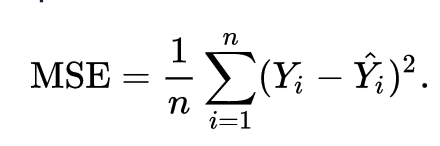# How Neural Networks Are Trained

Hey - Nick here! This page is a free excerpt from my \$199 course Python for Finance, which is 50% off for the next 50 students.

So far in this course, you have learned the following about neural networks:

We have not yet covered a very important part of the neural network engineering process: how neural networks are trained.

In this tutorial, you will learn how neural networks are trained. We'll discuss data sets, algorithms, and broad principles used in training modern neural networks that solve real-world problems.

## Hard-Coding vs. Soft-Coding

There are two main ways that you can develop computer applications. Before digging in to how neural networks are trained, it's important to make sure that you have an understanding of the difference between `hard-coding` and `soft-coding` computer programs.

Hard-coding means that you explicitly specify input variables and your desired output variables. Said differently, hard-coding leaves no room for the computer to interpret the problem that you're trying to solve.

Soft-coding is the complete opposite. It leaves room for the program to understand what is happening in the data set. Soft-coding allows the computer to develop its own problem-solving approaches.

A specific example is helpful here. Here are two instances of how you might identify cats within a data set using soft-coding and hard-coding techniques.

• Hard-coding: you use specific parameters to predict whether an animal is a cat. More specifically, you might say that if an animal's weight and length lie within certain
• Soft-coding: you provide a data set that contains animals labelled with their species type and characteristics about those animals. Then you build a computer program to predict whether an animal is a cat or not based on the characteristics in the data set.

As you might imagine, training neural networks falls into the category of soft-coding. Keep this in mind as you proceed through this course.

## Training A Neural Network Using A Cost Function

Neural networks are trained using a `cost function`, which is an equation used to measure the error contained in a network's prediction.

The formula for a deep learning cost function (of which there are many - this is just _one _example) is below:Note: this cost function is called the `mean squared error`, which is why there is an MSE on the left side of the equal sign.

While there is plenty of formula mathematics in this equation, it is best summarized as follows:

`Take the difference between the predicted output value of an observation and the actual output value of that observation. Square that difference and divide it by 2.`

Note that this is simply one example of a cost function that could be used in machine learning (although it is admittedly the most popular choice). The choice of which cost function to use is a complex and interesting topic on its own. We'll learn more about why this choice of cost function is so popular in our next section when we begin discussing gradient descent.

As mentioned, the goal of an artificial neural network is to minimize the value of the cost function. The cost function is minimized when your algorithm's predicted value is as close to the actual value as possible. Said differently, the goal of a neural network is to minimize the error it makes in its predictions!

## Modifying A Neural Network

After an initial neural network is created and its cost function is imputed, changes are made to the neural network to see if they reduce the value of the cost function.

More specifically, the actual component of the neural network that is modified is the weights of each neuron at its synapse that communicate to the next layer of the network.

The mechanism through which the weights are modified to move the neural network to weights with less error is called `gradient descent`. Gradient descent is a complicated topic and will be discussed in more detail in the next section of the course. For now, it's enough for you to understand that the process of training neural networks looks like this:

• Initial weights for the input values of each neuron are assigned
• Predictions are calculated using these initial values
• The predictions are fed into a cost function to measure the error of the neural network
• A gradient descent algorithm changes the weights for each neuron's input values
• This process is continued until the weights stop changing (or until the amount of their change at each iteration falls below a specified threshold)

This may seem very abstract - and that's ok! You will learn more about how neural networks are trained in the next section of this course.

## Final Thoughts

In this tutorial, you received a basic, no-code introduction to how deep learning neural networks are built and trained.